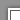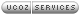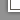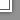TechSapphireSaturday, 2023-12-09, 8:00 AM Main | Introduction to the C Language | Registration | LoginWelcome Guest | RSS
 News
 Play Games Deep Freeze Ice Slide Gyroball Fat Fish Bush Royal Rampage

## THE FIRST PROGRAM IN C

### Introduction

The C program is a set of functions. The program execution begins by executing the function main (). You can compile the program and execute using Turbo C compiler or using the following commands in Unix/Linux:

```\$ cc -o a a.c
```

where a.c is the file in which you have written the program. This will create an executable file named a.exe.

```\$./a.
```

This will execute the program.

#### Program

```#include <stdio.h>
main()
{
printf("Hello \n"); /* prints Hello on standard output */ }

Output : Hello
```

#### Explanation

1. The program execution begins with the function main().

2. The executable statements are enclosed within a block that is marked by ‘{’ and ‘}’.

3. The printf() function redirects the output to a standard output, which in most cases is the output on screen.

4. Each executable statement is terminated by ‘;’

5. The comments are enclosed in ‘/*...*/

### Variables

#### Introduction

When you want to process some information, you can save the values temporarily in variables. In the following program you can define two variables, save the values, and put the addition in the third variable.

#### Program

```#include <stdio.h>
main()
{
int i,j,k; // Defining variables Statement A
i = 6; // Statement B
j = 8;
k = i + j;
printf("sum of two numbers is %d \n",k); // Printing results
}
output : sum of two numbers is 14
```

#### Explanation

1. Statement A defines variables of the type integer. For each variable you have to attach some data type. The data type defines the amount of storage allocated to variables, the values that they can accept, and the operations that can be performed on variables.

2. The ‘ // ’ is used as single line comment.

3. The ‘%d’ is used as format specifier for the integer. Each data type has a format specifier that defines how the data of that data type will be printed.

4. The assignment operator is ‘=’ and the statement is in the format:

Var = expression;

#### Points to Remember

1. The variables are defined at the begining of the block.

2. The data type is defined at the begining of declaration and followed by a list of variables.

3. It is the data type that assigns a property to a variable.

## INPUTTING THE DATA

#### Introduction

In C, the input from a standard input device, such as a keyboard, is taken by using the function scanf. In scanf, you have to specify both the variables in which you can take input, and the format specifier, such as %d for the integer.

#### Program

```#include <stdio.h>
main()
{
int i,j,k;
scanf("%d%d",&i,&j); // statement A
k = i + j;
printf("sum of two numbers is %d \n",k);
}
Input 3 4
Output: sum of two numbers is 7
```

#### Explanation

1. Statement A indicates the scanf statement that is used for taking input. In scanf you have to specify a list of addresses of variables (&i, &j) which can take input. Before specifying the list of variables you have to include a list of format specifiers that indicate the format of the input. For example, for the integer data type the format specifier is %d.

2. In scanf, you have to specify the address of the variable, such as &i. The address is the memory location where a variable is stored. The reason you must specify the address will be discussed later.

3. The number of format specifiers must be the same as the number of variables.

#### Point to Remember

In scanf, you have to specify the address of a variable, such as &i, &j, and a list of format specifiers.

## THE CONTROL STATEMENT (if STATEMENT)

### Introduction

You can conditionally execute statements using the if or the if...else statement. The control of the program is dependent on the outcome of the Boolean condition that is specified in the if statement.

#### Program

```#include <stdio.h>
main()
{
int i,j,big; //variable declaration
scanf("%d%d",&i,&j); big = i;
if(big < j) // statement A
{ // C
big = j; // Part Z, then part
} // D
printf("biggest of two numbers is %d \n",big);
if(i < j) // statement B
{
big = j; // Part X
}
else
{
big = i; // Part Y
}
printf("biggest of two numbers(using else) is %d \n",big);
}
```

#### Explanation

1. Statement A indicates the if statement. The general form of the if statement is if(expr)

```{
s1 ;
s2 ;
....
}
```
2. expr is a Boolean expression that returns true (nonzero) or false (zero).

3. In C, the value nonzero is true while zero is taken as false.

4. If you want to execute only one statement, opening and closing braces are not required, which is indicated by C and D in the current program.

5. The else part is optional. If the if condition is true then the part that is enclosed after the if is executed (Part X). If the if condition is false then the else part is executed (Part Y).

6. Without the else statement (in the first if statement), if the condition is true then Part Z is executed.

#### Points to Remember

1. if and if...else are used for conditional execution. After the if statement the control is moved to the next statement.

2. If the if condition is satisfied, then the "then" part is executed; otherwise the else part is executed.

3. You can include any operators such as <, >, <=, >=, = = (for equality). Note that when you want to test two expressions for equality, use = = instead of =.

## THE ITERATION LOOP (for LOOP)

### Introduction

When you want to execute certain statements repeatedly you can use iteration statements. C has provided three types of iteration statements: the for loop, while loop, and do...while loop. Generally, the statements in the loop are executed until the specified condition is true or false.

#### Program

```
#include <stdio.h>
main()
{
int i,n; //the
scanf("%d",&n);
for(i = 0; i<n; i= i+1) // statement A
{
printf("the numbers are %d \n",i); // statement B
}
}
/* input and output
5
the numbers are 0
the numbers are 1
the numbers are 2
the numbers are 3
the numbers are 4
*/
```

#### Explanation

1. Statement A indicates the for loop. The statements in the enclosing braces, such as statement B, indicate the statements that are executed repeatedly because of the for loop.

2. The format of the for loop is

```for (expr1;expr2;expr3)
{
s1;
s2 ; // repeat section
}
```
3. expr2 is a Boolean expression. If it is not given, it is assumed to be true.

4. The expressions expr1, expr2 and expr3 are optional.

5. expr1 is executed only once, the first time the for loop is invoked.

6. expr2 is executed each time before the execution of the repeat section.

7. When expr2 is evaluated false, the loop is terminated and the repeat section is not executed.

8. After execution of the repeat section, expr3 is executed. Generally, this is the expression that is used to ensure that the loop will be terminated after certain iterations.

#### Points to Remember

1. The for loop is used for repeating the execution of certain statements.

2. The statements that you want to repeat should be written in the repeat section.

3. Generally, you have to specify any three expressions in the for loop.

4. While writing expressions, ensure that expr2 is evaluated to be false after certain iterations; otherwise your loop will never be terminated, resulting in infinite iterations.

## THE do...while LOOP

### Introduction

The do...while loop is similar to the while loop, but it checks the conditional expression only after the repetition part is executed. When the expression is evaluated to be false, the repetition part is not executed. Thus it is guaranteed that the repetition part is executed at least once.

```Program
#include <stdio.h>
main()
{
int i,n; //the
scanf("%d",&n);
i = 0;
do // statement A
{
printf("the numbers are %d \n",i);
i = i +1;
}while( i<n) ;

}
/*
5
the numbers are 0
the numbers are 1
the numbers are 2
the numbers are 3
the numbers are 4
*/
```

#### Explanation

1. Statement A indicates the do...while loop.

2. The general form of the do...while loop is

```do
{
repetition part;
} while (expr);
```

When the do...while loop is executed, first the repetition part is executed, and then the conditional expression is evaluated. If the conditional expression is evaluated as true, the repetition part is executed again. Thus the condition is evaluated after each iteration. In the case of a normal while loop, the condition is evaluated before making the iteration.

3. The loop is terminated when the condition is evaluated to be false.

#### Point to Remember

The do...while loop is used when you want to make at least one iteration. The condition should be checked after each iteration.

## THE switch STATEMENT

### Introduction

When you want to take one of a number of possible actions and the outcome depends on the value of the expression, you can use the switch statement. switch is preferred over multiple if...else statements because it makes the program more easily read.

#### Program

```#include <stdio.h>
main()
{
int i,n; //the
scanf("%d",&n); for(i = 1; i<n; i= i+1)
{
switch(i%2) // statement A
{
case 0 : printf("the number %d is even \n",i); // statement B
break; // statement C
case 1 : printf("the number %d is odd \n",i);
break;
}
}
}
/*
5
the number 1 is odd
the number 2 is even
the number 3 is odd
the number 4 is even
*/
```

#### Explanation

The program demonstrates the use of the switch statement.

1. The general form of a switch statement is

```Switch(switch_expr)
{
case constant expr1 : S1;
S2;
break;
case constant expr1 : S3;
S4;
break;
.....
default : S5;
S6;
break;
}
```
2. When control transfers to the switch statement then switch_expr is evaluated and the value of the expression is compared with constant_expr1 using the equality operator.

3. If the value is equal, the corresponding statements (S1 and S2) are executed. If break is not written, then S3 and S4 are executed. If break is used, only S1 and S2 are executed and control moves out of the switch statement.

4. If the value of switch_expr does not match that of constant_expr1, then it is compared with the next constant_expr. If no values match, the statements in default are executed.

5. In the program, statement A is the switch expression. The expression i%2 calculates the remainder of the division. For 2, 4, 6 etc., the remainder is 0 while for 1, 3, 5 the remainder is 1. Thus i%2 produces either 0 or 1.

6. When the expression evaluates to 0, it matches that of constant_expr1, that is, 0 as specified by statement B, and the corresponding printf statement for an even number is printed. break moves control out of the switch statement.

7. The clause ‘default’ is optional.

#### Point to Remember

The switch statement is more easily read than multiple if...else statements and it is used when you want to selectively execute one action among multiple actions.

 Copyright MyCorp © 2023